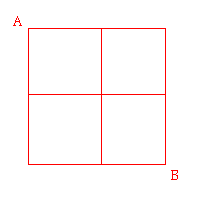## Thursday, June 26, 2008

### Dogs, Math, and Path Traversal

Every time I walk my dog, I like to take a different route. Here is my local neighborhood, showing the two or three block radius I walk around my house while walking the dog.I start and return to my house. Aside from my house, I do not traverse the same vertex twice. The two vertices at either end of my short block count as my house for this purpose; after leaving one of them, if I hit either one of them, I go home.

I didn't think there were that many unique paths I could walk. Turns out I was wrong.

Path Traversal

Much has been written on graph theory and path traversal algorithms, including finding all paths through all nodes, the shortest path between two nodes, and so on. I couldn't find my exact problem using a quick google.

I want to know how many unique paths there are between two nodes, where paths cannot visit the same vertex twice. (A more complicated question would be where you cannot visit the same path segment twice.)

Here's a simple graph:To get from A to B, there are 12 distinct paths you can take, without crossing the same vertex twice:
SSEE
SSENES
SSENNESS
SESE
SEES
SENESS
ESWSEE
ESSE
ESES
EESWWSEE
EESWSE
EESS
That's already more than the number of vertices, and equal to the number of path segments.

For a 2x2 grid, there are only 2 paths. For a 2x3, there are 4 paths. For 3x3 there are 12 paths. The number grows quickly:
2x2 = 2
3x3 = 12
4x4 = 184
5x5 = 8512
As I mentioned, if you can repeat vertices, but not paths, the number inflates much quicker:
2x2 = 2
3x3 = 16
4x4 = 800
5x5 = 323,632
Yowza.

My son, Saarya, wrote some basic code for this (I amended a little):
`#include<iostream>using namespace std;#define N 5#define M 5int cnt,tx,ty,lev;int mat[N][M];void print(){    for(int i=0;i<N;i++)    {        for(int j=0;j<M;j++) {             cout<<" ";             if(mat[i][j]<=9) {                   cout<<0;             }             cout<<mat[i][j];        }        cout<<endl;        cout<<"\n";    }    cout<<"------------------------------\n";       }    void go(int x, int y){          mat[x][y]=++lev;          if(x==tx&&y==ty)    {/*print();*/         cnt++;        mat[x][y]=0;        lev=lev-1;         return;     }        if(y-1>=0&&!mat[x][y-1])    {        go(x,y-1);    }        if(x-1>=0&&!mat[x-1][y])    {        go(x-1,y);    }        if(y+1<M&&!mat[x][y+1])    {        go(x,y+1);    }        if(x+1<N&&!mat[x+1][y])    {        go(x+1,y);    }    mat[x][y]=0;    lev=lev-1;}int main(){    for(int i=0;i<N;i++)        for(int j=0;j<M;j++)                mat[i][j]=0;    cnt=0;    tx=N-1;    ty=M-1;    go(0,0);    cout<<endl<<"-----------------------\n"<<cnt<<endl;    system("PAUSE");}      `

For path segments, Saarya's approach is to add extra matrix elements for the paths between each vertex and move two spaces each move.

Which all means that I could walk the rest of my life with my dog in a couple block radius and never take the same path twice.

Yehuda

#### 4 comments:

Ozvortex said...

Another advantage to one not walking the same route twice is it makes it more difficult for potential kidnappers and assassins. :)Anonymous said...

That's the famous Pet traversal problem...

David Klein said...

This can be done for general graphs with a slightly modified DFS. To quote from http://www.nist.gov/dads/HTML/allSimplePaths.html

"Note: The paths may be enumerated with a depth-first search. The search can avoid repeating vertices by marking them as they are visited in the recursion, then removing the mark just before returning from the recursive call."

For more details, see the link on that page.

Note: I believe the problem of counting the number of simple paths is NP complete (hard?). I.e. one can't do much better than just enumerating them as in the DFS above. See for example http://books.google.com/books?id=fyTk5AwnnoQC&pg=PT738&lpg=PT738&dq=counting+all+simple+paths+in+a+graph&source=web&ots=Xd0Adchszi&sig=C_aH_l8YW0wAeUJWyT67yejdyA8&hl=en&sa=X&oi=book_result&resnum=4&ct=result

Yehuda Berlinger said...

Thanks, David.

Yehuda